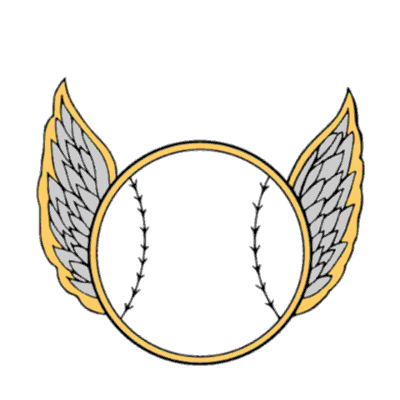# How do I update the location of a feature in an AGOL hosted Feature Layer?

345
1
06-11-2018 08:28 AMbyEsri Contributor

I am trying to randomly move a feature in an AGOL layer. The layer has a geometry property that is in web mercator and lat/long (WGS84) fields in the attribute table. Anyone know how I can update the location of the feature AND keep the lat/long fields in sync?

This is what I have tried:

``````latitude = feature.get_value("lat")
longitude = feature.get_value("long")
geometry = feature.get_value("geometry")

print (f"The latitude is {latitude}")
print (f"The longitude is {longitude}")
print (f"The geometry is {geometry}")

feature.set_value("lat", feature.get_value("lat")+random.uniform(0,1))
feature.set_value("long", feature.get_value("long")+random.uniform(0,1))

latitude = feature.get_value("lat")
longitude = feature.get_value("long")
geometry = feature.get_value("geometry")

print (f"The latitude is {latitude}")
print (f"The longitude is {longitude}")
print (f"The geometry is {geometry}")

geometry_dict = feature.get_value("geometry")
geometry_dict['x'] += random.uniform(0,10)
geometry_dict['y'] += random.uniform(0,10)
feature.set_value("geometry", geometry_dict)

print (f"The latitude is {latitude}")
print (f"The longitude is {longitude}")
print (f"The geometry is {geometry}")

'''
The code above results in the following output:

The latitude is 43.8849
The longitude is -100.3152
The geometry is {'x': -11167036.982825391, 'y': 5447647.436134641}
The latitude is 44.38470494318065
The longitude is -99.40659382285271
The geometry is {'x': -11167036.982825391, 'y': 5447647.436134641}
The latitude is 44.38470494318065
The longitude is -99.40659382285271
The geometry is {'x': -11167031.21161315, 'y': 5447647.819034746}

'''
‍‍‍‍‍‍‍‍‍‍‍‍‍‍‍‍‍‍‍‍‍‍‍‍‍‍‍‍‍‍‍‍‍‍‍‍‍‍‍‍‍‍``````

As you can see, changing the geometry does NOT change the Lat/Long properties. Do I have to do a conversion and update these fields separately? What would be the most efficient way to do this?by``````# WGS 1984 : (4326) Lat/Lon